# Filter (mathematics)

In set theory, a filter is a family of subsets of a given set which has properties generalising those of neighbourhoods in topology.

Formally, a filter on a set X is a subset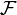$\mathcal{F}$ of the power set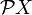$\mathcal{P}X$ with the properties:

1.$X \in \mathcal{F} ; \,$
2.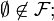$\empty \not\in \mathcal{F} ; \,$
3.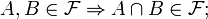$A,B \in \mathcal{F} \Rightarrow A \cap B \in \mathcal{F} ; \,$
4.$A \in \mathcal{F} \mbox{ and } A \subseteq B \Rightarrow B \in \mathcal{F} . \,$

If G is a nonempty subset of X then the family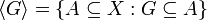$\langle G \rangle = \{ A \subseteq X : G \subseteq A \} \,$

is a filter, the principal filter generated by G.

In a topological space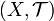$(X,\mathcal{T})$, the neighbourhoods of a point x$\mathcal{N}_x = \{ N \subseteq X : \exists U \in \mathcal{T} \;\, x \in U \subseteq N \} \,$

form a filter, the neighbourhood filter of x.

### Filter bases

A base$\mathcal{B}$ for the filter$\mathcal{F}$ is a non-empty collection of non-empty sets such that the family of subsets of X containing some element of$\mathcal{B}$ is precisely the filter$\mathcal{F}$.

## Ultrafilters

An ultrafilter is a maximal filter: that is, a filter on a set which is not properly contained in any other filter on the set. Equivalently, it is a filter$\mathcal{F}$ with the property that for any subset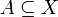$A \subseteq X$ either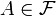$A \in \mathcal{F}$ or the complement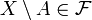$X \setminus A \in \mathcal{F}$.

The principal filter generated by a singleton set {x}, namely, all subsets of X containing x, is an ultrafilter.Some content on this page may previously have appeared on Citizendium.